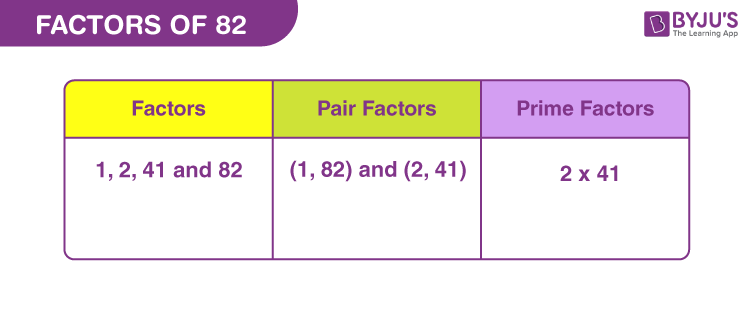# Factors of 82

Factors of 82 are the natural numbers that can evenly divide the original number. 82 is an even number, so it is divisible by 2 (by divisibility rules). If a number is even then that does not mean, it is only divisible by even numbers but also by odd numbers.

Factors are the one that break the number into equal numbers of parts. It is possible that a number can have any number of factors. 82 is a composite number that has more than two factors. Now let us learn the article to find more factors of 82 along with pair factors and prime factors.## How to Find the Factors of 82?

We can find the factors of 82 with easy methods. Either we can divide 82 by natural numbers to get the result as a whole number or we can multiply two numbers to get the result as 82.

 Division Method Multiplication Method 82 ÷ 1 = 82 1 x 82 = 82 82 ÷ 2 = 41 2 x 41 = 82 82 ÷ 41 = 2 41 x 2 = 82 82 ÷ 82 = 1 82 x 1 = 82

Therefore, the factors of 82 are 1, 2, 41 and 82.

## Pair Factors of 82

Pair factors of 82 are calculated by multiplying two integers, such that the product is equal to 82.

• 1 × 82 = 82
• 2 × 41 = 82

Therefore, the pair factors of 82 are (1, 82) and (2, 41).

If we consider the negative pair factors, then two negative integers will result in positive value after multiplication.

• -1 × -82 = 82
• -2 × -41 = 82

Therefore, the negative pair factors are (-1, -82) and (-2, -41).

## Prime Factorisation of 82

Prime factorisation of 82 will produce the prime numbers that can divide 82, uniformly. The prime factor form of 82 is the product of all the prime factors of 82, that results in the original number.

 Dividing 82 by smallest prime number 82 ÷ 2 = 41 41 is a prime number and is divisible by itself 41 ÷ 41 = 1

Therefore, the prime factors of 82 are 2 and 41.

 Prime factorisation of 82 = 2 x 41 Exponential form = 21 x 411

Also check: Prime Numbers

## Solved Examples

Q.1: Smita baked 82 cookies. She wants to distribute these cookies to 41 poor children. How many cookies does each child get?

Solution: Number of cookies = 82

Number of children = 41

Therefore, each child will get two cookies.

Q.2: What is the sum of all the factors of 82?

Solution: The factors of 82 are 1, 2, 41 and 82.

Sum = 1 + 2 + 41 + 82 = 126

Therefore, the required sum is 126.

Q.3: What are the common factors of 82 and 100?

Solution: We have to list the factors of 100 and 82.

82 → 1, 2, 41, 82

100 → 1, 2, 4, 5, 10, 20, 25, 50, and 100

Therefore, the common factors of 82 and 100 are 1 and 2.

Q.4: What is the LCM of 82 and 100?

Solution: We need to find the prime factorisation of both the numbers to find the LCM

82 → 2 x 41

100 → 2 x 2 x 5 x 5

Thus, LCM = 2 x 2 x 5 x 5 x 41

LCM (82, 100) = 4100

### Practice Questions

1. Evaluate the factors of 82 and write them in descending order.
2. Which factor of 82 is a prime number?
3. Find if 4 is a factor of 82.
4. What is the GCF of 72 and 82?

## Frequently Asked Questions on Factors of 82

### What are the factors of 82?

The factors of 82 are 1, 2, 41 and 82.

### What is the prime factor form of 82?

The prime factorisation of 82 is 2 x 41

### What are the multiples of 82?

The first ten multiples of 82 are 82, 164, 246, 328, 410, 492, 574, 656, 738 and 820.

### Is 82 a prime number or composite number?

82 is a composite number because it has more than two factors.

### Which is the second highest factor of 82?

The second highest factor of 82 is 41.